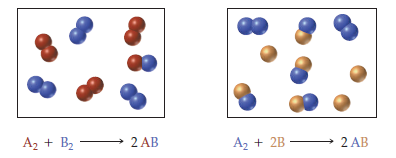×
Get Full Access to Fundamentals Of General, Organic, And Biological Chemistry (Mastering Chemistry) - 8 Edition - Chapter 7.9 - Problem 7.14
Get Full Access to Fundamentals Of General, Organic, And Biological Chemistry (Mastering Chemistry) - 8 Edition - Chapter 7.9 - Problem 7.14

×ISBN: 9780134015187 2044

## Solution for problem 7.14 Chapter 7.9

Fundamentals of General, Organic, and Biological Chemistry (Mastering Chemistry) | 8th Edition

• Textbook Solutions
• 2901 Step-by-step solutions solved by professors and subject experts
• Get 24/7 help from StudySoup virtual teaching assistantsFundamentals of General, Organic, and Biological Chemistry (Mastering Chemistry) | 8th Edition

4 5 1 237 Reviews
20
5
Problem 7.14

The following diagrams represent two similar reactions that have achieved equilibrium:Step-by-Step Solution:
Step 1 of 3

Angel Carrasquillo Week of 01/17/16­01/24/16 Chemistry 132 Notes ● Thermodynamics ­ If q is positive, reaction is endothermic. ­ If q is negative, reaction is exothermic. ● Constant Pressure ­ ΔEsystem = qp + w = qp + (­PΔv)(101.3J/L*atm) ­ ΔE const. pressure = ΔEconst. volume ­ At Const. Volume ΔE = qv ● Enthalpy ­ heat that’s released under const. pressure situations. ­ ΔG = ΔH ­ TΔS ­ ΔH = ΔE ­ w → ΔH = qp + w ­ w → ΔH = qp ● Calorimetry ­ how energy flow is measured. (ΔH) ­ open calorimeter, change in volume occurs (Δ V) ­ closed calorimeter, no Δ V only qv ● Open calorimeter = constant pressure ● Specific Heat Capacity (Cs) = J/K*gram ● Molar Heat Capacity (Cn) = J/K*mol ● q solution = ­q reaction ● ΔH reaction = ­q solution ­ reaction is endothermic, solution has increase in temperature ● q surroundings (solution) = nCnΔT or mCsΔT….n for mols, m for mass ● ΔV = Δ nRT/P ● When we have an expansion, work value is negative ● Molar heat capacity of an ideal gas ­ KE = 3/2RT ­ ΔKE = 3/2RΔT ­ Cv = 3/2R Cp = 5/2R (Cn for volume and pressure for ideal gases) ● heating gas results in loss of energy as work ● ΔEv = ΔEp ­ ΔE = nCvΔT = qv ­ ΔE = nCpΔ T + w qp = nCpΔT = ΔH ● For real molecules, Cv > 3/2R, Cp > 5/2R ● Heats of Formation ­ ΔHf Knot = standard conditions (

Step 2 of 3

Step 3 of 3

## Discover and learn what students are asking

Calculus: Early Transcendental Functions : First-Order Linear Differential Equations
?In Exercises 1-4, determine whether the differential equation is linear. Explain your reasoning. $$\frac{2-y^{\prime}}{y}=5 x$$

Unlock Textbook Solution